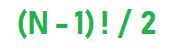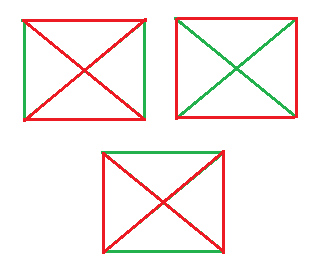# Number of Hamiltonian cycle

Given an undirected complete graph of N vertices where N > 2. The task is to find the number of different Hamiltonian cycle of the graph.

Complete Graph: A graph is said to be complete if each possible vertices is connected through an Edge.

Hamiltonian Cycle: It is a closed walk such that each vertex is visited at most once except the initial vertex. and it is not necessary to visit all the edges.

Formula:Examples:

```Input : N = 6
Output : Hamiltonian cycles = 60

Input : N = 4
Output : Hamiltonian cycles = 3
```

## Recommended: Please try your approach on {IDE} first, before moving on to the solution.

Explanation:
Let us take the example of N = 4 complete undirected graph, The 3 different hamiltonian cycle is as shown below:Below is the implementation of the above approach:

## C++

 `// C++ program for implementation of the  ` `// above program ` ` `  `#include ` `using` `namespace` `std; ` ` `  `// Function that calculates ` `// number of Hamiltonian cycle ` `int` `Cycles(``int` `N) ` `{ ` ` `  `    ``int` `fact = 1, result = 0; ` ` `  `    ``result = N - 1; ` ` `  `    ``// Calculating factorial ` `    ``int` `i = result; ` `    ``while` `(i > 0) { ` `        ``fact = fact * i; ` `        ``i--; ` `    ``} ` ` `  `    ``return` `fact / 2; ` `} ` ` `  `// Driver code ` `int` `main() ` `{ ` `    ``int` `N = 5; ` ` `  `    ``int` `Number = Cycles(N); ` ` `  `    ``cout << ``"Hamiltonian cycles = "` `<< Number; ` `    ``return` `0; ` `} `

## Java

 `// Java program for implementation  ` `// of the above program ` `class` `GFG ` `{ ` `     `  `// Function that calculates ` `// number of Hamiltonian cycle ` `static` `int` `Cycles(``int` `N) ` `{ ` `    ``int` `fact = ``1``, result = ``0``; ` ` `  `    ``result = N - ``1``; ` ` `  `    ``// Calculating factorial ` `    ``int` `i = result; ` `    ``while` `(i > ``0``)  ` `    ``{ ` `        ``fact = fact * i; ` `        ``i--; ` `    ``} ` ` `  `    ``return` `fact / ``2``; ` `} ` ` `  `// Driver code ` `public` `static` `void` `main(String[] args) ` `{ ` `    ``int` `N = ``5``; ` ` `  `    ``int` `Number = Cycles(N); ` ` `  `    ``System.out.println(``"Hamiltonian cycles = "` `+ ` `                                        ``Number); ` `} ` `} ` ` `  `// This code is contributed  ` `// by Code_Mech. `

## Python3

 `# Python3 program for implementation  ` `# of the above program ` `import` `math as mt ` ` `  `# Function that calculates ` `# number of Hamiltonian cycle ` `def` `Cycles(N): ` ` `  `    ``fact ``=` `1` ` `  `    ``result ``=` `N ``-` `1` ` `  `    ``# Calculating factorial ` `    ``i ``=` `result ` `    ``while` `(i > ``0``): ` `        ``fact ``=` `fact ``*` `i ` `        ``i ``-``=` `1` `     `  `    ``return` `fact ``/``/` `2` ` `  `# Driver code ` `N ``=` `5` ` `  `Number ``=` `Cycles(N) ` ` `  `print``(``"Hamiltonian cycles = "``,  ` `                       ``Number) ` ` `  `# This code is contributed ` `# by Mohit Kumar `

## C#

 `// C# program for implementation of  ` `// the above program ` `using` `System; ` ` `  `class` `GFG ` `{ ` ` `  `// Function that calculates ` `// number of Hamiltonian cycle ` `static` `int` `Cycles(``int` `N) ` `{ ` `    ``int` `fact = 1, result = 0; ` ` `  `    ``result = N - 1; ` ` `  `    ``// Calculating factorial ` `    ``int` `i = result; ` `    ``while` `(i > 0)  ` `    ``{ ` `        ``fact = fact * i; ` `        ``i--; ` `    ``} ` ` `  `    ``return` `fact / 2; ` `} ` ` `  `// Driver code ` `public` `static` `void` `Main() ` `{ ` `    ``int` `N = 5; ` ` `  `    ``int` `Number = Cycles(N); ` ` `  `    ``Console.Write(``"Hamiltonian cycles = "` `+ ` `                                   ``Number); ` `} ` `} ` ` `  `// This code is contributed  ` `// by Akanksha Rai `

## PHP

 ` 0) ` `    ``{  ` `        ``\$fact` `= ``\$fact` `* ``\$i``;  ` `        ``\$i``--;  ` `    ``}  ` ` `  `    ``return` `floor``(``\$fact` `/ 2);  ` `}  ` ` `  `// Driver code  ` `\$N` `= 5;  ` ` `  `\$Number` `= Cycles(``\$N``);  ` ` `  `echo` `"Hamiltonian cycles = "``, ` `                     ``\$Number``;  ` ` `  `// This code is contributed by Ryuga ` `?> `

Output:

```Hamiltonian cycles = 12
```

My Personal Notes arrow_drop_upCheck out this Author's contributed articles.

If you like GeeksforGeeks and would like to contribute, you can also write an article using contribute.geeksforgeeks.org or mail your article to contribute@geeksforgeeks.org. See your article appearing on the GeeksforGeeks main page and help other Geeks.

Please Improve this article if you find anything incorrect by clicking on the "Improve Article" button below.